Courses

# Concentration Formulae Class 11 Notes | EduRev

## JEE : Concentration Formulae Class 11 Notes | EduRev

The document Concentration Formulae Class 11 Notes | EduRev is a part of JEE category.
All you need of JEE at this link: JEE

Concentration Formulae

There are several methods to express the concentration of solutions. We are going to describe some very important terms used to represent the concentration of solutions.

• The percent by Weight (w/w): It is the weight of the solute as a percent of the total weight of the solution.
% by weight of solute = Weight of solute/Weight of solution × 100
Example: If a solution of HCI contains 36 % HCI by weight, it has 36 g of HCI in 100 g of solution.
• The percent by Volume (v/v): It is the volume of solute present per 100 ml of solution.
% by Volume = Volume of solute/Volume of solution × 100
Example: If we have 35% C2H5OH solution by volume means 35 ml C2H5OH is present per 100 ml of the solution.
• Percent by weight/volume (w/v): It is the mass of solute present per 100g of solution.
% = Weight of solution/Volume of solution × 100
Example: 30% HCl solution, means 30 g of HCl is present per 100 ml of solution.
• Mole Fraction (X):  A simple solution is made of two substances: One is the solute and the other is solvent. Mole fraction, X, of solute is defined as the ratio of the number of moles of solute and the total number of moles of solute and solvent.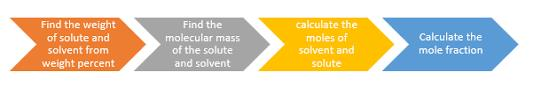Thus,
Xsolute = Moles of solute/Moles of solute + Moles of solvent
If n represents moles of solute and N number of moles of solvent, Then
Xsolute = n/n + N
Notice that mole fraction of solvent would be
Xsolvent = n/n + N
Mole fraction is unitless and (Xsolute + Xsolvent) = 1
• Molarity (M): In current practice, concentration is most often expressed as molarity. Molarity is defined as the number of moles of solute per litre of solution.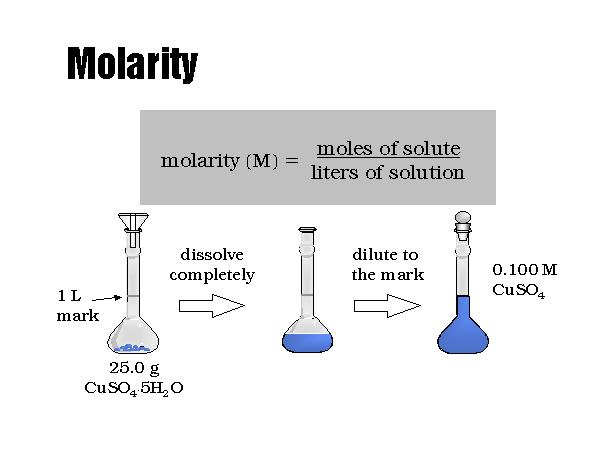Molarity = Moles of solute/Volume (solution) in litres
or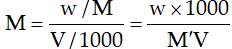w = Mass of solute in grams.
M`= Molecular weight of solute in gm/mol.
V = Volume of solution in ml.
0.01 M NaOH, solution means that 0.01 mole NaOH is present in 1000 ml of its solution.
• Molality (m): Molality of a solution is defined as the number of moles of solute per kilogram of solvent.
Molality = Moles of solute/Mass of solvent in kilograms
or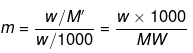where
w = mass of solute in grams.
M`= molecular wt of solute in gm/mol.
W = mass of solvent in grams.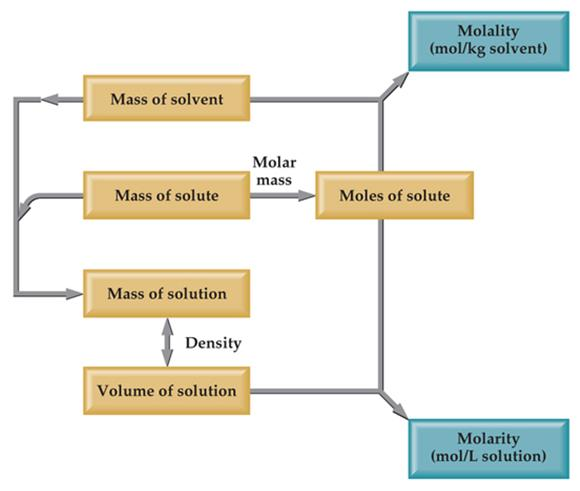• Normality (N): Normality of a solution is defined as the number of equivalents of solute per litre of the solution.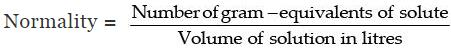or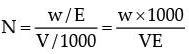w = Mass of solute in gram.
V = Volume of solution in ml.
E = Equivalent wt. of solute.
• ppm (parts per million): It is the mass of solute in grams present per 106 grams of solution.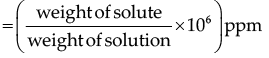and also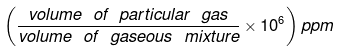Often in a chemistry laboratory, a solution of the desired concentration is prepared by diluting a solution of known higher concentration. The solution of higher concentration is also known as a stock solution. Note that molality of a solution does not change with temperature since mass remains unaffected with temperature.

Example 1. A solution is prepared by adding 2 g of substance A to 18 g of water. Calculate the mass percent of the solute.
Solution.
Mass per cent
= 2/(2 g of A + 18g)
= 2 g/20 g x 100
= 10 %

Example 2. Calculate the molarity of NaOH in the solution prepared by dissolving its 4 g in enough water to form 250 mL of the solution
Solution.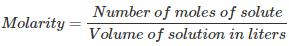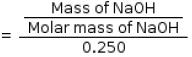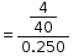= 0.4

Example 3. The density of 3 M solution of NaCl is 1.25 g mL–1. Calculate the molality of the solution.
Solution.

M = 3 mol L–1
Mass of NaCl in 1 L solution = 3 × 58.5 = 175.5 g
Mass of 1 L solution = Mass of solution × density of solution
= 1000 × 1.25
= 1250 g
(since density = 1.25 g mL–1)
Mass of water in solution = 1250 –175.5 = 1074.5 g
Molality = mass of solute in number of moles/mass of solvent in kg
= 3/1.07468 kg
= 2.7915 Molal

Example 4. A solution of NaCl 0.5% by wt. If the density of the solution is 0.997 g/ml, calculate
(a) The molality
(b) Molarity
(c) Normality
(d) Mole fraction of the solute

Solution.
Number of moles of NaCl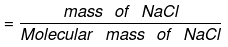= 0.5/58.5 = 0.00854

(a) By definition,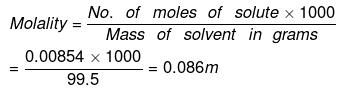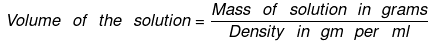= 100/0.997 = 100.3
(b) Now Molarity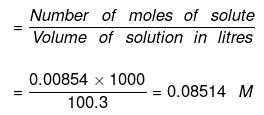(c) Normality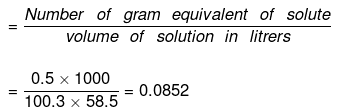(d) To calculate mole fraction of the solute
No. of moles of water in 99.5 g = 99.5/18 = 5.5277
Moles of NaCl = 0.5/58.5 = 8.547 x 10-3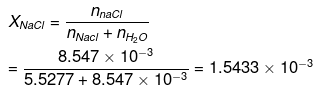XH2O = 1 - XNaCI = 0.9984

Example 5. Calculate the molarity of a solution containing 0.5 g of NaOH dissolved in  500 cm3.
Solution. Weight of NaOH dissolved = 0.5 g
Volume of the solution = 500 cm3
Calculation of molarity:
0.5 g of NaOH = 0.5/40 mole of NaOH
(∴ Mol. mass of NaOH = 40)
= 0.0125 mole
Thus 500 cm3 of solution contain NaOH = 0.0125 mole
∴ 1000 cm3 of the solution contain NaOH
= 0.0125/500 = 1000
⇒ 0.025 mole
Hence, molarity of the solution = 0.025 M

Example 6. Calculate the molarity of a solution containing 9.8 gm of H2SO4 in 250 cm3 of the solution.
Solution. Mass of H2SO4 dissolved = 9.8 g
Volume of the solution = 250 cm3
Calculation of molarity
Mol. mass of H2SO4 = 98
∴ No. of moles of H2SO4 = Mass in g/Mol. mass
= 9.8/98 = 10
250 cm3 of the solution contain H2SO4
= 0.1 mole
∴ 1000 cm3 of the solution contain H2SO4
= 0.1/250 x 1000 = 0.4 mole
Hence molarity of solution = 0.4 M

Example 7. Find the molarity and molality of a 15% solution of H2SO4 (density of H2SO= 1.020 g cm− 3) (Atomic mass: H = 1, O = 16, S = 32 amu).
Solution.
15% of a solution of H2SO4 means 15 g of H2SO4 are present in 100 g of the solution i.e.
Mass of H2SO4 dissolved = 15 g
Mass of the solution = 100 g
Density of the solution = 1.02 g/cm3 (given)
Calculation of molality:
Mass of solution = 100 g
Mass of H2SO4 = 15g
Mass of water (solvent) = 100 – 15 = 85 g
Mol. mass of H2SO4 = 98
∴ 15 g H2SO4 = 15/98 = 0.153 moles
Thus 85 g of the solvent contain 0.153 moles 100 g of the solvent containing
= 0.153/85 × 1000 = 1.8
Hence the molality of H2SO4 solution = 1.8 m
Calculation of molarity:
15 g of H2SO4 = 0.153 moles
Vol. of solution = W t. of solution/Density of solution = 100/1.02 = 98.04 cm3
Thus 98.04 cmof solution contain H2SO4 = 0.153 moles

Try Yourself!

Q.1. The density of 3M solution of Na2S2O3 is 1.25g ml–1. Calculate
(a) The % by weights of Na2S2O
(b) Mole fraction of Na2S2O3
(c) The molalities of Na+ and S2O3−2 ions
Solution:

Ans. (a) 37.92
(a) Mass of 1000 mL of Na2S2O3 solution = 1.25×1000=1250g
Mass of Na2S2O3 in 1000 mL of 3 M solution
=3×MolmassofNa2S2O3
=3×158=474g
Mass percentage of Na2S2O3 in solution
= 474/1250  X 100= 37.92 %

(b) mole fraction of Na2S2O3
Ans. (b) 0.065
Solution:
(ii) No. of moles of Na2S2O3 = 474/158= 3
Mass of water =(1250−474)=776g
No. of moles of water =  776/18 =43.1
Mole fraction of Na2S2O3 = 3/43.1+3  =0.065

(c) the molalities of Na+ and S2O3−2 ions.
Ans: (c) 3.865
Solution:
No. of moles of Na+ ions
=2×No.of moles of Na2S2O3
=2×3=6
Molality of Na+ ions =No.of moles of Na+ ions/Mass of water in kg
= 6/776 X 1000
=7.73m
No. of moles of S2O32− ions = No. of mole of Na2S2O3
=3
Molality of S2O32−−ions =3/776 X 1000=3.86m Na2S2O3

Q.2. A sample of H2SO4 (density 1.787g ml–1) is labelled as 86% by weight. What is molarity of acid? What volume of acid has to be used to make 1 litre of 0.2M H2SO4?
Ans. 12.75 ml
Solution: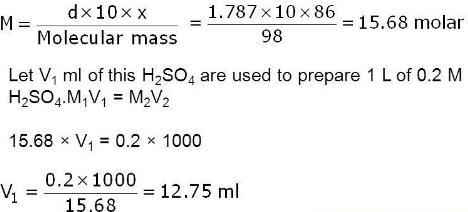Q.3. What weight of AgCl will be precipitated when a solution containing 4.77 g NaCl is added to a solution of 4.77 g of AgNO3?
Ans. 4.87 g
Solution: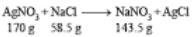From the given data, we find AgNO3 is limiting reagent as NaCl is in excess.
∵ 170.0 g of AgNOprecipitated AgCl = 143.5 g
∴ 5.77 g of AgNOprecipitated AgCl
= 143.5/170.0 x 5.77 = 4.87 g

Q.4. How much BaCl2 would be needed to make 250 ml of a solution having same concentration of Cl as the one containing 3.78 g of NaCl per 100 ml?
Ans. 16.80
Solution:
Moles of NaCl=  3.78/58.5=0.0646
1 NaCl contains one Cl
∴ moles of Cl− ions =0.0646
∴ (Cl−)= no. of moles/Volume in litres = 0.064615/0.1
∴ concentration of Cl⁻ = [Cl⁻] = 0.646
Again, BaCl ⇄ Ba⁺ + 2Cl⁻
∴ [BaCl] = [Ba⁺] = [Cl⁻]/2
From above concentration of Cl⁻= 0.646 M
∴ [BaCl] = [Cl⁻]/2 = 0.646/2 = 0.323M
Now, molarity of BaCl = weight of BaCl₂/molar weight of BaCl × volume in L 0.323 = weight of BaCl/(208.3 × 0.25)
[ ∵ molar weight of BaCl2 = 208.3g/mol and volume of solution = 250ml = 0.25L
∴0.323 × 208.3 × 0.25 = weight of BaCl2
weight of BaCl2 = 16.8g
Hence, weight of BaCl = 16.8g

Offer running on EduRev: Apply code STAYHOME200 to get INR 200 off on our premium plan EduRev Infinity!

,

,

,

,

,

,

,

,

,

,

,

,

,

,

,

,

,

,

,

,

,

;